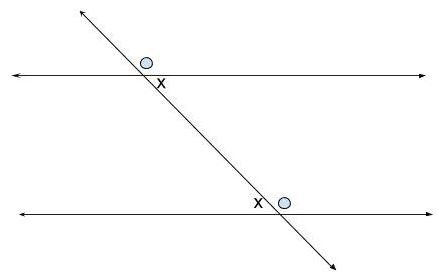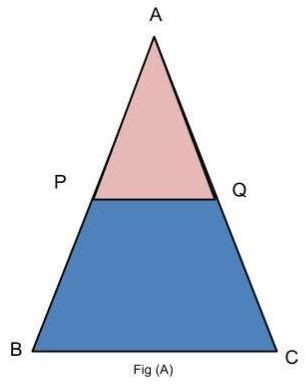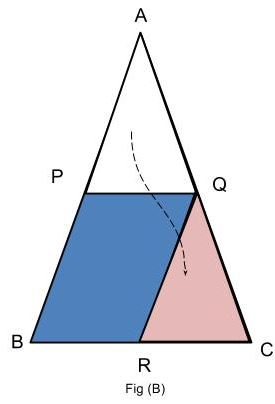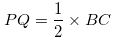# Procedure - To verify the mid-point theorem for a triangle, Math, Class 9 Class 9 Notes | EduRev

## Class 9 : Procedure - To verify the mid-point theorem for a triangle, Math, Class 9 Class 9 Notes | EduRev

The document Procedure - To verify the mid-point theorem for a triangle, Math, Class 9 Class 9 Notes | EduRev is a part of the Class 9 Course Mathematics (Maths) Class 9.
All you need of Class 9 at this link: Class 9

Pre-requisite knowledge:

Two lines are parallel if for a transversal cutting them, the corresponding angles are equal.Procedure:

As performed in the real lab:

Materials Required:

Colored papers, sketch pens, geometry box, a pair of scissors, fevicol and eraser.

Procedure:

1. Form a sheet of paper.

2. Cut a ▲ABC.

3. Find Mid-points P and Q of AB and AC respectively by paper folding.

4. Join P and Q by folding and making a crease PQ.

5. Cut ▲APQ.

6. Superimpose AQ over QC so that QP falls along CB.

As performed in the simulator:

Procedure:

1. Create ▲ABC by providing length of each side AB,BC and AC in dimension box.

2. Mark mid-point of each line AB,BC,AC as P,Q,R respectively.

3. Now join PQ and QR.

4. Click on cut triangle button to get replicate triangle of APQ.

5. Drag this replica and place it at ▲ QRC.Observation:

1. Line PQ || Line BC

2. PQ=RC

3.Result:

“The line segment connecting the midpoints of  two sides of a triangle is parallel to the third side and is congruent to one half of the third side. ”

Offer running on EduRev: Apply code STAYHOME200 to get INR 200 off on our premium plan EduRev Infinity!

## Mathematics (Maths) Class 9

190 videos|233 docs|82 tests

,

,

,

,

,

,

,

,

,

,

,

,

,

,

,

,

,

,

,

,

,

,

,

,

,

,

,

;